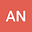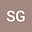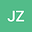A mathematical formalism for circulation in water mass configuration space
•••• +1## Abstract

We present a mathematical formalism for water mass analysis and circulation by formulating mass continuity, tracer continuity, circulation streamfunction, and tracer angular momentum within water mass configuration space (q-space), which is defined by an arbitrary number of continuous properties. Points in geometric position space (x-space) do not generally correspond in a 1-to-1 manner to points in q-space. We therefore formulate q-space as a differentiable manifold, which allows for differential and integral calculus but lacks a metric, with the use of exterior algebra and exterior calculus enabling us to develop q-space mass and tracer budgets. The Jacobian, which measures the ratio of volumes in x-space and q-space, is central to our theory. When x-space is not 1-to-1 with q-space, we define a generalized Jacobian either by patching together x-space regions that are 1-to-1 with q-space, or by integrating a Dirac delta to select all x-space points corresponding to a given q value. The latter method discretizes to a binning algorithm, thus providing a practical framework for water mass analysis. Considering q-space defined by tracers, we show that diffusion is directly connected to local tracer space circulation and angular momentum. We also show that diffusion, remarkably, cannot change globally integrated tracer angular momentum (unless different tracers are diffused differently, as in double diffusion), thus leaving only boundary processes (e.g., air–sea or land–sea fluxes), or interior sources to generate globally integrated tracer angular momentum.